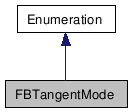# FBTangentMode Class Reference

FBTangentMode FBTangentMode FBTangentMode FBTangentMode FBTangentMode FBTangentMode FBTangentMode FBTangentMode FBTangentMode FBTangentMode FBTangentMode FBTangentMode FBTangentMode FBTangentMode FBTangentMode FBTangentMode FBTangentMode FBTangentMode FBTangentMode FBTangentMode FBTangentMode FBTangentMode FBTangentMode FBTangentMode FBTangentMode FBTangentMode FBTangentMode FBTangentMode FBTangentMode FBTangentMode FBTangentMode FBTangentMode FBTangentMode FBTangentMode FBTangentMode FBTangentMode FBTangentMode FBTangentMode FBTangentMode FBTangentMode FBTangentMode FBTangentMode FBTangentMode FBTangentMode FBTangentMode FBTangentMode FBTangentMode FBTangentMode FBTangentMode FBTangentMode FBTangentMode FBTangentMode FBTangentMode FBTangentMode FBTangentMode FBTangentMode FBTangentMode FBTangentMode FBTangentMode FBTangentMode FBTangentMode FBTangentMode FBTangentMode FBTangentMode FBTangentMode FBTangentMode FBTangentMode FBTangentMode FBTangentMode FBTangentMode FBTangentMode FBTangentMode FBTangentMode FBTangentMode FBTangentMode FBTangentMode FBTangentMode FBTangentMode FBTangentMode FBTangentMode FBTangentMode FBTangentMode FBTangentMode FBTangentMode FBTangentMode FBTangentMode FBTangentMode FBTangentMode FBTangentMode FBTangentMode FBTangentMode FBTangentMode FBTangentMode FBTangentMode FBTangentMode FBTangentMode FBTangentMode FBTangentMode FBTangentMode FBTangentMode FBTangentMode FBTangentMode FBTangentMode FBTangentMode FBTangentMode FBTangentMode FBTangentMode FBTangentMode FBTangentMode FBTangentMode FBTangentMode FBTangentMode FBTangentMode FBTangentMode FBTangentMode FBTangentMode FBTangentMode FBTangentMode FBTangentMode FBTangentMode
Inheritance diagram for FBTangentMode:[legend]

List of all members.

## Detailed Description

Methods of tangent calculation.

This is only relevant when interpolation is CUBIC.

## Public Attributes

kFBTangentModeAuto
This is the equivalent to a cardinal spline with no parametrization.
kFBTangentModeTCB
TCB spline (3 parameters: TENSION, CONTINUITY, BIAS).
kFBTangentModeUser
Used to represent all splines with no lost data (HERMITE, BEZIER, CATMUL, etc.).
kFBTangentModeBreak
Like USER but left slope may differ from right.

## Member Data Documentation

This is the equivalent to a cardinal spline with no parametrization.

TCB spline (3 parameters: TENSION, CONTINUITY, BIAS).

Used to represent all splines with no lost data (HERMITE, BEZIER, CATMUL, etc.).

Like USER but left slope may differ from right.

Generated on Tue Feb 9 19:34:40 2010 for Python Reference Guide by1.5.3